# Priority Queue

In this tutorial, you will learn what priority queue is. Also, you will find working examples of priority queue operations in C, C++, Java and Python.

A priority queue is a special type of queue in which each element is associated with a priority and is served according to its priority. If elements with the same priority occur, they are served according to their order in the queue.

Generally, the value of the element itself is considered for assigning the priority.

For example: The element with the highest value is considered as the highest priority element. However, in other case, we can assume the element with the lowest value as the highest priority element. In other cases, we can set priority according to our need.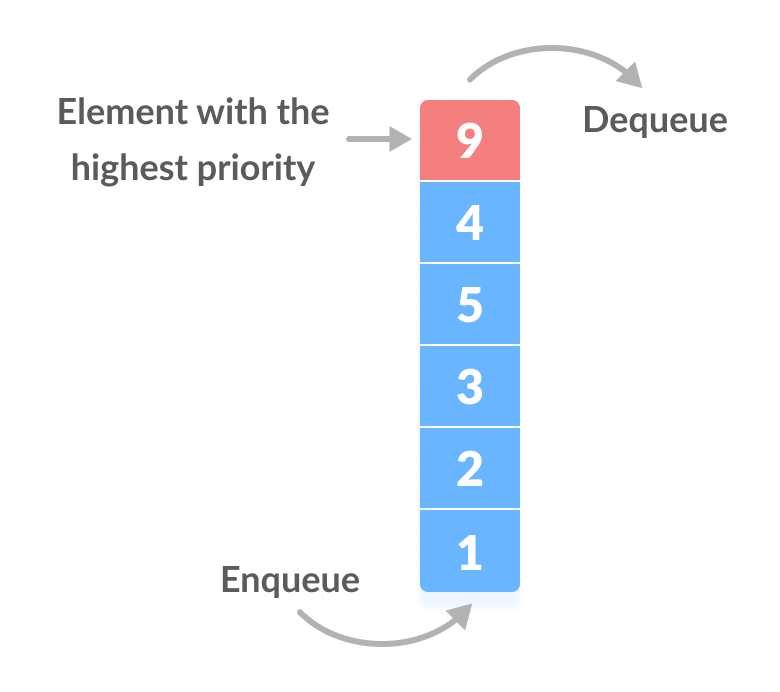How priority queue differs from a queue?

In a queue, the first-in-first-out rule is implemented whereas, in a priority queue, the values are removed on the basis of priority. The element with the highest priority is removed first.

## Implementation of Priority Queue

Priority queue can be implemented using an array, a linked list, a heap data structure or a binary search tree. Among these data structures, heap data structure provides an efficient implementation of priority queues.

A comparative analysis of different implementations of priority queue is given below.

peek insert delete
Linked List `O(1)` `O(n)` `O(1)`
Binary Heap `O(1)` `O(log n)` `O(log n)`
Binary Search Tree `O(1)` `O(log n)` `O(log n)`

## Priority Queue Operations

Basic operations of a priority queue are inserting, removing and peeking elements.

Before studying the priority queue, please refer to the heap data structure for better understanding of binary heap as it is used to implement priority queue in this article.

### Inserting an Element from the Priority Queue

Inserting an element into a priority queue (max heap) is done by following steps.

1. Insert the new element at the end of the tree.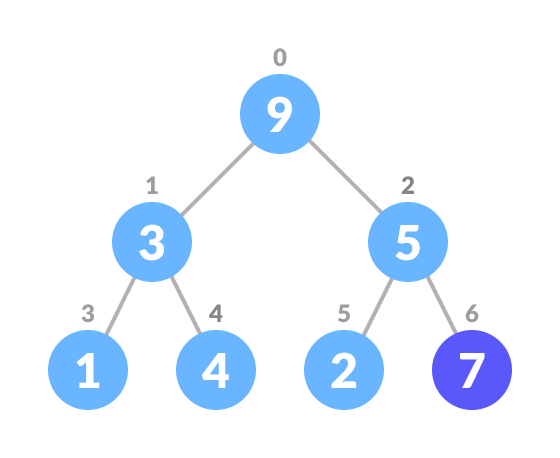2. Heapify the tree.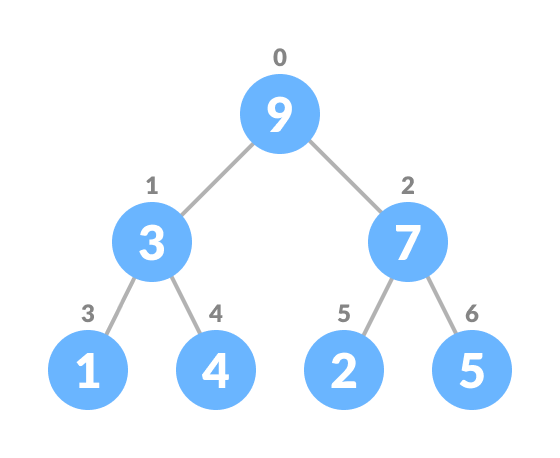Algorithm for insertion of an element into priority queue (max-heap)

``````If there is no node,
create a newNode.
else (a node is already present)
insert the newNode at the end (last node from left to right.)

heapify the array``````

For Min Heap, the above algorithm is modified so that `parentNode` is always smaller than `newNode`.

### Deleting an Element from the Priority Queue

Deleting an element from a priority queue (max heap) is done as follows:

1. Select the element to be deleted.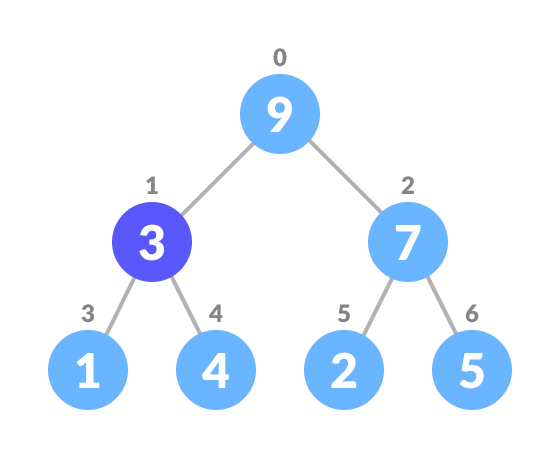2. Swap it with the last element.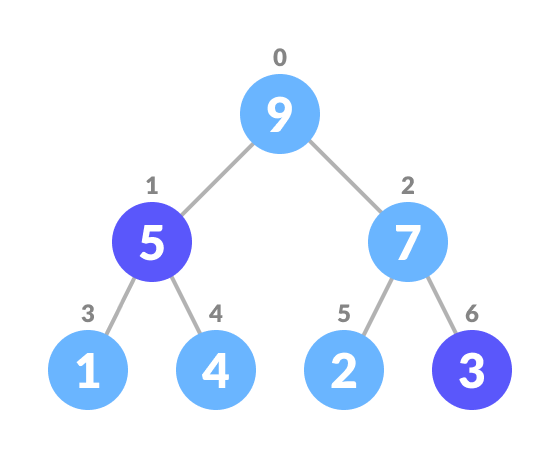3. Remove the last element.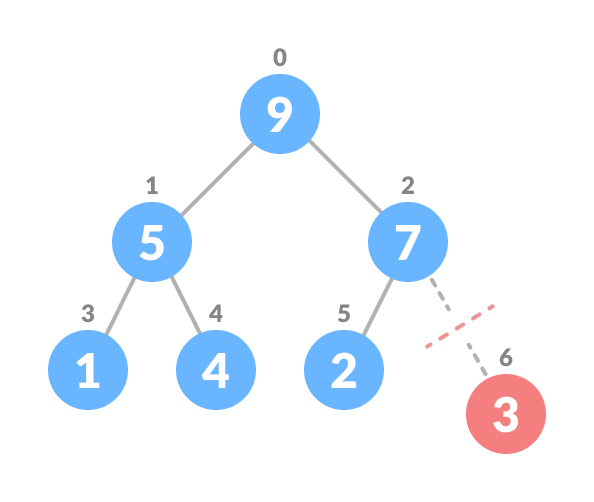4. Heapify the tree.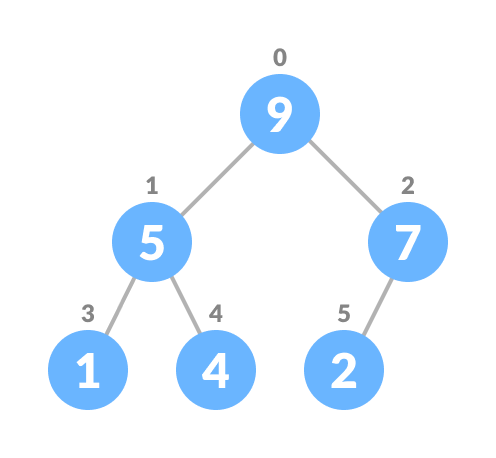Algorithm for deletion of an element in the priority queue (max-heap)

``````If nodeToBeDeleted is the leafNode
remove the node
Else swap nodeToBeDeleted with the lastLeafNode
remove noteToBeDeleted

heapify the array``````

For Min Heap, the above algorithm is modified so that the both `childNodes` are greater smaller than `currentNode`.

### Peeking from the Priority Queue (Find max/min)

Peek operation returns the maximum element from Max Heap or minimum element from Min Heap without deleting the node.

For both Max heap and Min Heap

`return rootNode`

### Extract-Max/Min from the Priority Queue

Extract-Max returns the node with maximum value after removing it from a Max Heap whereas Extract-Min returns the node with minimum value after removing it from Min Heap.

## Python, Java, C/C++ Examples

``````# Max-Heap data structure in Python

def heapify(arr, n, i):
largest = i
l = 2 * i + 1
r = 2 * i + 2

if l < n and arr[i] < arr[l]:
largest = l

if r < n and arr[largest] < arr[r]:
largest = r

if largest != i:
arr[i],arr[largest] = arr[largest],arr[i]
heapify(arr, n, largest)

def insert(array, newNum):
size = len(array)
if size == 0:
array.append(newNum)
else:
array.append(newNum);
for i in range((size//2)-1, -1, -1):
heapify(array, size, i)

def deleteNode(array, num):
size = len(array)
i = 0
for i in range(0, size):
if num == array[i]:
break

array[i], array[size-1] = array[size-1], array[i]

array.remove(size-1)

for i in range((len(array)//2)-1, -1, -1):
heapify(array, len(array), i)

arr = []

insert(arr, 3)
insert(arr, 4)
insert(arr, 9)
insert(arr, 5)
insert(arr, 2)

print ("Max-Heap array: " + str(arr))

deleteNode(arr, 4)
print("After deleting an element: " + str(arr))

``````
``````
// Max-Heap data structure in Java

import java.util.ArrayList;

class Heap {
void heapify(ArrayList<Integer> hT, int i) {
int size = hT.size();
int largest = i;
int l = 2 * i + 1;
int r = 2 * i + 2;
if (l < size && hT.get(l) > hT.get(largest))
largest = l;
if (r < size && hT.get(r) > hT.get(largest))
largest = r;

if (largest != i) {
int temp = hT.get(largest);
hT.set(largest, hT.get(i));
hT.set(i, temp);

heapify(hT, largest);
}
}

void insert(ArrayList<Integer> hT, int newNum) {
int size = hT.size();
if (size == 0) {
} else {
for (int i = size / 2 - 1; i >= 0; i--) {
heapify(hT, i);
}
}
}

void deleteNode(ArrayList<Integer> hT, int num)
{
int size = hT.size();
int i;
for (i = 0; i < size; i++)
{
if (num == hT.get(i))
break;
}

int temp = hT.get(i);
hT.set(i, hT.get(size-1));
hT.set(size-1, temp);

hT.remove(size-1);
for (int j = size / 2 - 1; j >= 0; j--)
{
heapify(hT, j);
}
}

void printArray(ArrayList<Integer> array, int size) {
for (Integer i : array) {
System.out.print(i + " ");
}
System.out.println();
}

public static void main(String args[]) {

ArrayList<Integer> array = new ArrayList<Integer>();
int size = array.size();

Heap h = new Heap();
h.insert(array, 3);
h.insert(array, 4);
h.insert(array, 9);
h.insert(array, 5);
h.insert(array, 2);

System.out.println("Max-Heap array: ");
h.printArray(array, size);

h.deleteNode(array, 4);
System.out.println("After deleting an element: ");
h.printArray(array, size);
}
}``````
``````// Max-Heap data structure in C

#include <stdio.h>
int size = 0;
void swap(int *a, int *b)
{
int temp = *b;
*b = *a;
*a = temp;
}
void heapify(int array[], int size, int i)
{
if (size == 1)
{
printf("Single element in the heap");
}
else
{
int largest = i;
int l = 2 * i + 1;
int r = 2 * i + 2;
if (l < size && array[l] > array[largest])
largest = l;
if (r < size && array[r] > array[largest])
largest = r;
if (largest != i)
{
swap(&array[i], &array[largest]);
heapify(array, size, largest);
}
}
}
void insert(int array[], int newNum)
{
if (size == 0)
{
array = newNum;
size += 1;
}
else
{
array[size] = newNum;
size += 1;
for (int i = size / 2 - 1; i >= 0; i--)
{
heapify(array, size, i);
}
}
}
void deleteRoot(int array[], int num)
{
int i;
for (i = 0; i < size; i++)
{
if (num == array[i])
break;
}

swap(&array[i], &array[size - 1]);
size -= 1;
for (int i = size / 2 - 1; i >= 0; i--)
{
heapify(array, size, i);
}
}
void printArray(int array[], int size)
{
for (int i = 0; i < size; ++i)
printf("%d ", array[i]);
printf("\n");
}
int main()
{
int array;

insert(array, 3);
insert(array, 4);
insert(array, 9);
insert(array, 5);
insert(array, 2);

printf("Max-Heap array: ");
printArray(array, size);

deleteRoot(array, 4);

printf("After deleting an element: ");

printArray(array, size);
}``````
``````// Max-Heap data structure in C++

#include <iostream>
#include <vector>
using namespace std;

void swap(int *a, int *b)
{
int temp = *b;
*b = *a;
*a = temp;
}
void heapify(vector<int> &hT, int i)
{
int size = hT.size();
int largest = i;
int l = 2 * i + 1;
int r = 2 * i + 2;
if (l < size && hT[l] > hT[largest])
largest = l;
if (r < size && hT[r] > hT[largest])
largest = r;

if (largest != i)
{
swap(&hT[i], &hT[largest]);
heapify(hT, largest);
}
}
void insert(vector<int> &hT, int newNum)
{
int size = hT.size();
if (size == 0)
{
hT.push_back(newNum);
}
else
{
hT.push_back(newNum);
for (int i = size / 2 - 1; i >= 0; i--)
{
heapify(hT, i);
}
}
}
void deleteNode(vector<int> &hT, int num)
{
int size = hT.size();
int i;
for (i = 0; i < size; i++)
{
if (num == hT[i])
break;
}
swap(&hT[i], &hT[size - 1]);

hT.pop_back();
for (int i = size / 2 - 1; i >= 0; i--)
{
heapify(hT, i);
}
}
void printArray(vector<int> &hT)
{
for (int i = 0; i < hT.size(); ++i)
cout << hT[i] << " ";
cout << "\n";
}

int main()
{
vector<int> heapTree;

insert(heapTree, 3);
insert(heapTree, 4);
insert(heapTree, 9);
insert(heapTree, 5);
insert(heapTree, 2);

cout << "Max-Heap array: ";
printArray(heapTree);

deleteNode(heapTree, 4);

cout << "After deleting an element: ";

printArray(heapTree);
}``````

## Priority Queue Applications

Some of the applications of a priority queue are:

1. Dijkstra's algorithm
2. for implementing stack
3. for load balancing and interrupt handling in an operating system
4. for data compression in Huffman code